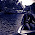## Friday, 21 June 2013

### Finding the longest matching pattern in a given input using FSM simulation

Finding a string in the input that matches a given pattern is a task accomplished by a Lexical Analyzer. LEX (a lexical analyzer generator) uses two disambiguation rules, one of which is to give more preference to the longer string in the input which matches a given pattern (in the form of a regular expression).This article simply explains how this is achieved in detail.

LEX converts the regular expressions given to it into a Finite Automaton, which is deterministic in nature. To see how this is done , visit  http://silcnitc.github.io/documentation.html. It has not been explained here because it will take us beyond the scope of the article.

The constructed DFA is then used to accept or reject a string in the input. Simply accepting a string when the automaton enters an accept state would not suffice, because this wouldn't correspond to the longest match, instead it would find the shortest match. The automaton must remember the last time it had entered the accepting state so that once the automaton enters a dead state, (A dead state is reached when the automaton has no further transitions from the current state on the provided input) it can simply backtrack to the last accepted state and accept the string which caused it to enter an accept state in the first place.

In this manner, the automaton will always look for a longer matching string (call it string A) in the input (even if a string B has already been matched). If A exists for the same pattern, then the automaton accepts that string. If there is no such string A, the automaton will simply end up in a dead state, and since it had kept track of the last accepted state, it simply backtracks to that state and accepts string B.

In order to achieve this, the automaton simulator program must keep track of two variables :

1. Last accepted state
2. Position of the input scanner at the last accepted state

The requirement for the latter of the two is so that the input scanner can resume scanning from this position if it encounters a dead state. i.e. It must resume scanning from after string B in the input. Hence now the scanner restarts scanning from this position, thus treating it as a new input string. This process continues till the end of the input is reached.

NOTE: String B is just a part of the input, not the entire input itself. Same applies for string A.

### How is this achieved ?  (Algorithm) :

/*NOTE :
->Here states are numbered {0,1,2....}
->Transition table is a double dimensional array indexed with states(rows) and inputs(columns) and resultant state stored in the elements.
-> ' \in ' is a LATEX notation for 'belongs to'
->'1' is the start state
->Accepting_states[ ] is an array of accepting states
*/
Last_acc_pos=0         //Last position at which the automaton reached the accepting state

Last_acc_state=0       //Last state which was accepted by the automaton
Current_pos=0           //Current position of the scanner

WHILE ( !EOF )    c=getNextChar_from( Current_pos )
Current_pos++
//Setting the current state of the machine
IF ( Last_acc_state != 0 )
Current_state = Transition[Last_acc_state,c]
ELSE
Current_state = Last_acc_state = 1
Current_pos--    //retract by one position because read was insignificant here
//Checking whether to accept and/or continue scanning
IF ( Current_state \in Accepting_states )
Last_acc_state=Current_state
Last_acc_pos=Current_pos
ELSE IF (Current_state = 0)
/*Print acceptance of string */
Last_acc_state=0
Current_pos=Last_acc_pos

1.2.